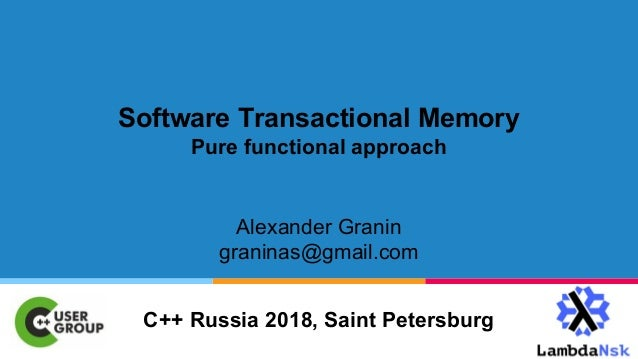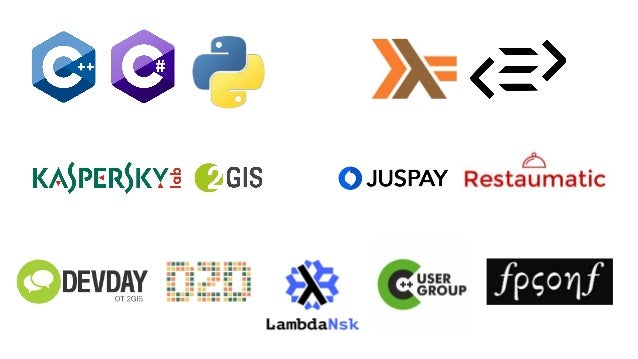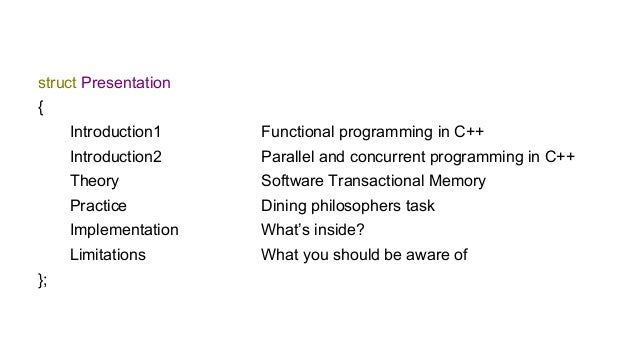Successfully reported this slideshow.You’ve finished this document.
Upcoming SlideShare
Next

ofUpcoming SlideShare
Next

Share

# Software transactional memory. pure functional approach

Slides for C++ Russia 2018
I'm presenting my `cpp_stm_free` library: composable monadic STM for C++ on Free monads for lock-free concurrent programming.

See all

See all

### Software transactional memory. pure functional approach

1. 1. Software Transactional Memory Pure functional approach Alexander Granin graninas@gmail.com C++ Russia 2018, Saint Petersburg
2. 2. struct Presentation { Introduction1 Functional programming in C++ Introduction2 Parallel and concurrent programming in C++ Theory Software Transactional Memory Practice Dining philosophers task Implementation What’s inside? Limitations What you should be aware of };
3. 3. Introduction 1. Functional programming in C++ ● Lambdas, closures, functions (almost pure) ● std::function<> ● Combining of pure functions ● Immutability, POD-types ● Templates - pure functional language ● for_each(), recursion ● Type inference ● Pain
4. 4. std::thread std::future std::promise std::async Introduction 2. Parallel and concurrent programming in C++ std::atomic std::mutex std::condition_variable
5. 5. Theory. Software Transactional Memory ● Concurrent data model ● Independent parallel computations (“transactions”) over a different parts of the model ● Atomic commit of changed at the end of the computations: ○ Successful, if there are no conflicts of competing changes ○ Failed, if there are conflicts ■ Changes will be rollbacked, transaction will be restarted ● It’s also nice to have: ○ Absence of explicit synchronizations ○ Composable transactions ○ Capability to retry transactions ○ Adequate behavior and safety
6. 6. ● New blocks: ○ atomic_noexcept ○ atomic_cancel ○ atomic_commit ○ synchronized ● Transaction-safe functions ○ transaction_safe ● Pros: ○ Transaction-safe functions ● Cons: ○ Too chaotic ○ Too far from release ○ Bad way to do STM ● Requirements: ○ GCC 6.1 Technical Specification ISO/IEC TS 19841:2015 int f() { static int i = 0; atomic_noexcept { ++i; return i; } }
7. 7. Wyatt-STM WSTM::Atomically ([&](WSTM::WAtomic& at) { ++repeat1; v1.Set (v1.Get (at) + v2.Get (at), at); barrier.wait (); }); ● Pros: ○ Composable transactions ○ Retry operation ○ In production ● Cons: ○ Too imperative: easy to hack ○ Has some unwanted weakness ○ Boost ● Requirements: ○ Visual Studio 2015, GCC 4.9, Clang 3.4 ○ Boost 1.57 ● https://github.com/bretthall/Wyatt-STM ● CppCon 2015: Brett Hall “Transactional Memory in Practice"
8. 8. My library: cpp_stm_free STML<ForkPair> readForks(const TForkPair& forks) { STML<Fork> lm = readTVar(forks.left); STML<Fork> rm = readTVar(forks.right); return both(lm, rm, mkForkPair); }; ● Pros: ○ Purely functional: hard to hack ○ Composable monadic transactions ○ Retry operation ○ Adequate behavior ○ Many ways to improve and optimize ● Cons: ○ Highly experimental, Proof of Concept ○ Mind blowing, probably ○ Performance - ??? (will measure later) ● Requirements: ○ GCC 7.2, C++17 ● https://github.com/graninas/cpp_stm_free
9. 9. TVar<int> tMyInt = newTVarIO(10); TVar operations
10. 10. TVar<int> tMyInt = newTVarIO(10); auto someResult = readTVar(tMyInt); TVar operations
11. 11. TVar<int> tMyInt = newTVarIO(10); auto someResult = readTVar(tMyInt); writeTVar(tMyInt, 20); TVar operations
12. 12. TVar<int> tMyInt = newTVarIO(10); auto someResult = readTVar(tMyInt); writeTVar(tMyInt, 20); // Why IO? // someResult is int? // Doesn’t return anything, right? TVar operations
13. 13. TVar<int> tMyInt = newTVarIO(10); STML<int> someResult = readTVar(tMyInt); STML<Unit> m = writeTVar(tMyInt, 20); Transactions STML<int> - transactional type, thus: readTVar - transaction (returns stm::STML<T>) writeTVar - transaction (returns stm::STML<Unit>) newTVarIO - not a transaction (returns something else) // Why IO? // → Not a transaction. // someResult is int? // → Nope. It’s STML<int>. // Doesn’t return anything, right? // → Actually returns STML<Unit>.
14. 14. template <typename A> STML<TVar<A>> newTVar(const A& val); template <typename A> STML<A> readTVar(const TVar<A>& tvar); template <typename A> STML<Unit> writeTVar(const TVar<A>& tvar, const A& val); template <typename A> STML<A> retry(); template <typename A> STML<A> pure(const A& val); Transactions newTVar :: A → STML<TVar<A>> readTVar :: TVar<A> → STML<A> writeTVar :: TVar<A> → A → STML<Unit> retry :: STML<A> pure :: A → STML<A>
15. 15. Composing transactions bind :: STML<A> → (A → STML<B>) → STML<B> transaction :: STML<int> transaction = do tvar ← newTVar (10) readTVar (tvar) template <typename A, typename B> STML<B> bind(const STML<A> ma, const function<STML<B>(A)>& f); STML<int> transaction() { STML<TVar<int>> t1 = newTVar(10); STML<int> t2 = bind(t1, [](const TVar<int>& tvar) { return readTVar(tvar); }); return t2; }
16. 16. STML<TVar<int>> t1 = newTVar(10); STML<int> t2 = bind(t1, readTVar); // short form without lambda Context context; // All TVars live in the context int result1 = atomically(context, t2); // result1 == 10 int result2 = atomically(context, t2); // result2 == 10, also* // * but can be different on case of many competing threads trying to change this TVar. Atomic evaluation
17. 17. STML<TVar<int>> t1 = newTVar(10); STML<int> t2 = bind(t1, readTVar); // short form without lambda STML<int> t3 = bind(t2, [](int i) { if (i == 10) return retry(); // retry if i == 10 return pure(i + 1); // return incremented i otherwise }); Context context; // All TVars live in the context int result1 = atomically(context, t2); // result1 == 10 int result2 = atomically(context, t2); // result2 == 10, also* int result3 = atomically(context, t3); // tries hard to retry the transaction // * but can be different on case of many competing threads trying to change this TVar. Retrying
18. 18. template <typename A, typename B, typename Ret> STML<Ret> both(const STML<A>& ma, const STML<B>& mb, const function<Ret(A, B)>& f); template <typename A, typename B> STML<B> sequence(const STML<A>& ma, const STML<B>& mb); template <typename B> STML<B> ifThenElse(const STML<bool>& m, const STML<B>& mOnTrue, const STML<B>& mOnFalse); Useful combinators // Do both computations separately, // combine the results with function, // return combined result // Do the first computation, // drop its result, // do the second computation, // return its result // Do the conditional computation `m`, // if true, do `mOnTrue` // otherwise, do `mOnFalse`
19. 19. Practice. Dining philosophers task
20. 20. enum class ForkState { Free, Taken }; struct Fork { std::string name; ForkState state; }; enum class Activity { Thinking, Eating }; struct TForkPair { TVar<Fork> left; TVar<Fork> right; }; struct Philosopher { std::string name; TVar<Activity> activity; TForkPair forks; };
21. 21. // Fine grained TVar<Fork> tFork1 = newTVarIO(someContext, Fork {"1", ForkState::Free}); TVar<Fork> tFork2 = newTVarIO(someContext, Fork {"2", ForkState::Free}); TVar<Fork> tFork3 = newTVarIO(someContext, Fork {"3", ForkState::Free}); // Coarse grained std::vector<Fork> forks = { Fork {"1", ForkState::Free} , Fork {"2", ForkState::Free} , Fork {"3", ForkState::Free} } TVar<std::vector<Fork>> tForks = newTVarIO(someContext, forks); Fine vs. Coarse Grained
22. 22. Taking a fork STML<bool> takeFork(const TVar<Fork>& tFork) { STML<bool> alreadyTaken = withTVar(tFork, isForkTaken); STML<Unit> takenByUs = modifyTVar(tFork, setForkTaken); STML<bool> success = sequence(takenByUs, pure(true)); STML<bool> fail = pure(false); STML<bool> result = ifThenElse(alreadyTaken, fail, success); return result; }; const function<bool(Fork)> isForkTaken = [](const Fork& fork) { return fork.state == ForkState::Taken; }; const function<Fork(Fork)> setForkTaken = [](const Fork& fork) { return Fork { fork.name, ForkState::Taken }; };
23. 23. Taking both forks STML<bool> takeForks(const TForkPair& forks) { STML<bool> leftTaken = takeFork(forks.left); STML<bool> rightTaken = takeFork(forks.right); STML<bool> bothTaken = both(leftTaken, rightTaken, [](bool l, bool r) { return l && r; }); return bothTaken; };
24. 24. Philosopher: changing activity STML<Activity> changeActivity(const Philosopher& philosopher) { STML<Activity> mActivity = readTVar(philosopher.activity); STML<Activity> mNewActivity = bind(mActivity, [=](Activity currentActivity) { if (currentActivity == Activity::Thinking) { STML<bool> taken = takeForks(philosopher.forks); // try take forks STML<Unit> change = writeTVar(philosopher.activity, Activity::Eating); STML<Unit> m = ifThenElse(taken, change, retry()); // retry if not taken return sequence(m, pure(Activity::Eating)); } // more code here } return mNewActivity; }
25. 25. Running the task void philosopherWorker(stm::Context& context, const Philosopher& philosopher) { while (true) { stm::atomically(context, changeActivity(philosopher)); std::this_thread::sleep_for(std::chrono::seconds(5)); } } stm::Context context; stm::TVar<Fork> tFork1 = stm::newTVarIO(context, Fork {"1", ForkState::Free }, " Fork 1"); stm::TVar<Fork> tFork2 = stm::newTVarIO(context, Fork {"2", ForkState::Free }, " Fork 2"); Philosopher philosopher1 = mkPhilosopher(context, "1", tFork1, tFork2); // default activity TVar Philosopher philosopher2 = mkPhilosopher(context, "2", tFork2, tFork1); // will be created here std::thread(philosopherWorker, context, philosopher1); std::thread(philosopherWorker, context, philosopher2);
26. 26.  (2) Eating, 1=Taken : 2=Taken  (1) Thinking, 2=Taken : 3=Taken  (2) Eating, 3=Taken : 4=Taken  (1) Thinking, 4=Taken : 5=Free  (1) Thinking, 5=Free : 1=Taken Philosopher 3 changed activity to: Thinking for 3 seconds. Philosopher 1 changed activity to: Thinking for 3 seconds. Philosopher 2 changed activity to: Eating for 1 seconds. Philosopher 4 changed activity to: Eating for 1 seconds.  (3) Thinking, 1=Free : 2=Taken  (2) Eating, 2=Taken : 3=Taken  (3) Thinking, 3=Taken : 4=Taken  (2) Eating, 4=Taken : 5=Taken  (1) Thinking, 5=Taken : 1=Free Sample console output
27. 27. Implementation. What’s inside?
28. 28. Embedded Domain-Specific Language for STM template <typename A, typename Next> struct NewTVar { A val; std::function<Next(TVar<A>)> next; }; template <typename A, typename Next> struct ReadTVar { TVar<A> tvar; std::function<Next(A)> next; }; template <typename A, typename Next> struct WriteTVar { TVar<A> tvar; A val; std::function<Next(fp::Unit)> next; }; template <typename A, typename Next> struct Retry { };
29. 29. STM Algebraic Data Type template <class Ret> struct STMF { std::variant<NewTVar <std::any, Ret>, ReadTVar <std::any, Ret>, WriteTVar <std::any, Ret>, Retry <std::any, Ret> > stmf; };
30. 30. template <typename Ret> struct PureF { Ret ret; }; template <typename Ret> struct FreeF { STMF<STML<Ret>> stmf; }; template <typename Ret> struct STML { std::variant<PureF<Ret>, FreeF<Ret> > stml; }; Free Monad Recursive Algebraic Data Type template <class Ret> struct STMF { std::variant<NewTVar <std::any, Ret>, ReadTVar <std::any, Ret>, WriteTVar <std::any, Ret>, Retry <std::any, Ret> > stmf; };
31. 31. Free Monad Recursive Algebraic Data Type STML<A>→ STML<A> → FreeF<A> → STMF<STML<A>> → ReadTVar<std::any, A> → [](std::any any) { return STML<A> → PureF<A> → std::any_cast<A>(any) }
32. 32. Free Monad Recursive Algebraic Data Type STML<A>→ STML<A> → FreeF<A> → STMF<STML<A>> → ReadTVar<std::any, A> → [](std::any any) { return STML<A> → PureF<A> → std::any_cast<A>(any) }
33. 33. Monadic binding template <typename A, typename B> struct BindStmlVisitor; template <typename A, typename B> struct BindStmfVisitor; template <typename A, typename B> STML<B> bind(const STML<A>& stml, const std::function<STML<B>(A)>& f) { BindStmlVisitor<A, B> visitor(f); std::visit(visitor, stml.stml); return visitor.result; }
34. 34. “Pattern-matching” template <typename A, typename B> struct BindStmfVisitor { void operator() (const NewTVar<std::any, STML<A>>& method); void operator() (const ReadTVar<std::any, STML<A>>& method); void operator() (const WriteTVar<std::any, STML<A>>& method); void operator() (const Retry <std::any, STML<A>>&); }; template <typename A, typename B> struct BindStmlVisitor { void operator() (const PureF<A>& variant); void operator() (const FreeF<A>& variant); };
35. 35. Typed / Untyped template <typename A, typename Next> struct ReadTVar { // ReadTVar<A, Next> TVar<A> tvar; // TVar<A> std::function<Next(A)> next; ReadTVar<std::any, Next> toAny() const { std::function<Next(A)> nextCopy = next; ReadTVar<std::any, Next> m; // ReadTVar<std::any, Next> m.tvar = TVar<std::any> { tvar.id }; // TVar<std::any> m.next = [=](const std::any& val) { // A → std::any A val2 = std::any_cast<A>(val); // std::any → A return nextCopy(val2); }; return m; } };
36. 36. TVar Interface & Implementation using TVarId = utils::GUID; template <typename T> struct TVar { std::string name; TVarId id; }; struct TVarHandle { GUID ustamp; std::any data; }; using TVars = std::map<TVarId, TVarHandle>; class Context { std::map<TVarId, TVarHandle> _tvars; std::mutex _lock; public: bool tryCommit(const GUID& ustamp, const TVars& stagedTvars); };
37. 37. Limitations. What you should be aware of ● Side effects in transactions are prohibited ● Proper binding of transactions ● Proper data model (fine grained vs coarse grained) ● Occasional infinite retry ● Dangerous closures (be careful passing things by reference) ● Starvation ● Deadlock / livelock ● Implementation isn’t optimal by performance (yet)
38. 38. Thanks for watching! Alexander Granin graninas@gmail.com C++ Russia 2018, Saint Petersburg
•Jan. 3, 2021

Total views

818

On Slideshare

0

From embeds

0

Number of embeds

0

7

Shares

0